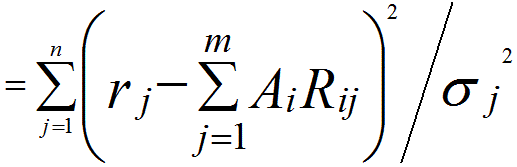Linear Least Squares Fitting Technique
- Practical Electron Microscopy and Database -
- An Online Book -
Microanalysis | EM Book                                                                                   https://www.globalsino.com/EM/

=================================================================================

Linear least squares fitting (LLSF) technique is the simplest and most commonly used form of linear regression and provides a solution to a problem of finding the best fitting straight line through a set of points.

There are many applications with this technique, for instance, in the quantifications in electron- and photon-induced X-rays, linear least-square fitting can be used to deconvolute the overlapped peaks once the continuum background is eliminated by a proper technique. In the LLSF method, it is assumed that each channel of the measured spectrum is equal to the sum of a set of the reference spectra in the same channel, as given below,
rj = Aj1•Rj1 + Aj2•Rj2 + Aj3•Rj3 + ... + Ajn•Rjn + Ej ---------------------- [1760a]---------------------------------- [1760b]
where,
j -- The channel j.
rj -- The measured signal in chanel j.
Aj1, Aj2, Rj3 ... Ajn -- The corresponding coefficients which are used to LLSF fitting.
Rj1, Rj2, Rj3 ... Rjn -- The signals of the reference spectra in chanel j.
Ej -- The statistical error which represents the degree of noise and fluctuation in channel j.
1, 2, ... n -- The pure elements.

The reference spectra may be any set of curves that can be extracted from a proper model, including the information of the shape and amplitude of the particular X-ray lines of interest. In general, such references are a set of measured spectra from the pure elements 1, 2, ... n. According to the least-squares method, the best set of the Ai coefficients are obtained by minimizing the value of x2, which is given by,------------------------------- [1760c]----------------------------- [1760d]
where,
x2 -- The Chi-Squared.
σj -- The standard deviation that represents the statistical uncertainty of rj.

In ideal least-squares fitting, if the x2 is normalized (dividing by the number of fitted points minus degrees of freedom), it should be approximately one in average. Values of x2 that are much greater than one indicates some systematic error in the fit.

Different from the ideal case described in Equation 1760b, the practical least-squares fitting must incorporate a treatment of the continuum differences. 

The procedure of fitting the spectrum from a sample using LLSF technique is:
i) Record spectra from standards to obtain reference spectra. The reference profiles can be experimentally determined using pure element or simple compound standards.
ii) Record an EDS profile from a sample, including the peaks of all the elements.
iii) Subtract background from the sample spectrum, either explicitly or by digital filtering with a zero-area correlator like the “Top-Hat” [2,3].
iv) Use linear least squares algorithm to find the best combination of profile intensities of the reference spectra to match the sample spectrum that gives the minimum sum of squares of differences with the background-subtracted sample spectrum discussed above.
v) Refine the analysis by giving more weight to those channels where the statistical standard deviation is small.
vi) Calculate the overall statistical precision of the determined intensities.

 McCarthy, J.J., and F.H. Schamber, 1981, Least-Squares Fit with Digital Filter: A Status Report.  In Energy Dispersive X-ray Spectrometry, edited by K.F.J. Heinrich, D.E. Newbury, R.L. Myklebust, and C.E. Fiori, pp. 273-296.  National Bureau of Standards Special Publication 604, Washington, D.C.
 F. H. Schamber, A modification of the linear least-squares fitting method which provides continuum suppression, Proc. Symp. X-Ray Fluorescence Analysis of Environmental Samples, Chapel Hill, N.C. 1976, T. Dzubay, ed., Ann Arbor Science Pub., Ann Arbor, Mich. (1977) pp. 241-257.
 P. J. Statham, Deconvolution and Background Subtraction by Least-Squares Fitting with Prefiltering of Spectra, Anal. Chem. 49, 2149-2154 (1977).

=================================================================================